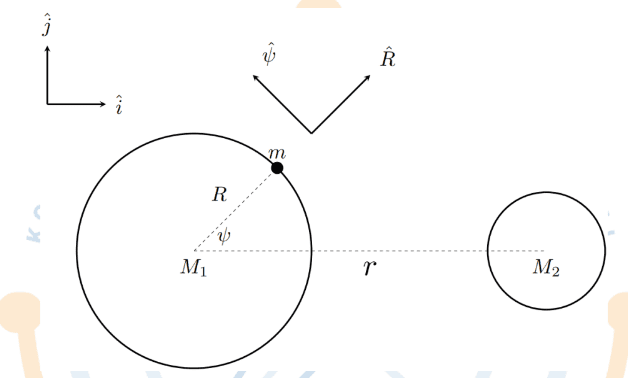# Fictitious force in a binary stars

Rikudo
Homework Statement:
Two planets with mass M1 and M2 revolve around each other in circular orbits. The distance between them is r. A mass m that is located on the surface of M1 with radius R feels fictitious force that modifies the mass's acceleration. Assume that mass m is very small and ignore the effect from M1's rotation. (see figure)

Write down the vector of the fictitious force in m, M2, r, gravity constant G, and unit vector!
Relevant Equations:
-I have a difficulty in understanding the question.
Fictitious force is a force whose motion is described using a non-inertial frame of reference. Which frame is the question referring to?

•